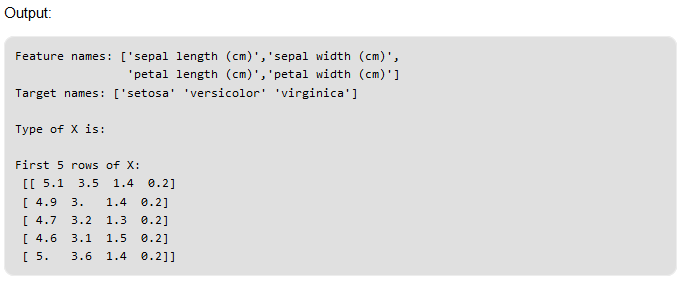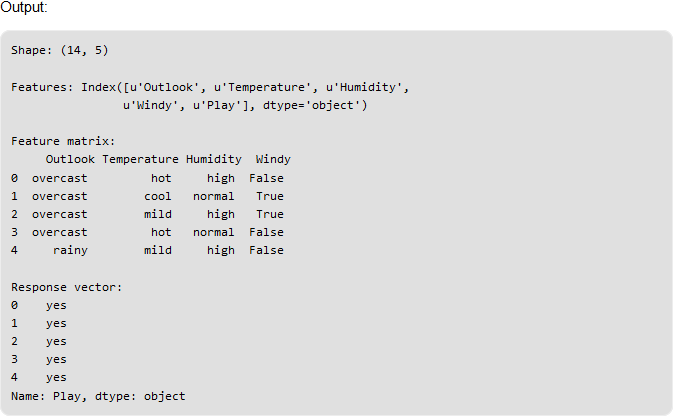# Chapter 15: Learning Model Building in Scikit-learn : A Python Machine Learning Library

Pre-requisite: Getting started with machine learning
scikit-learn is an open source Python library that implements a range of machine learning, pre-processing, cross-validation and visualization algorithms using a unified interface.

## Important features of scikit-learn:

• Simple and efficient tools for data mining and data analysis. It features various classification, regression and clustering algorithms including support vector machines, random forests, gradient boosting, k-means, etc.
• Accessible to everybody and reusable in various contexts.
• Built on the top of NumPy, SciPy, and matplotlib.
• Open source, commercially usable – BSD license.

In this article, we are going to see how we can easily build a machine learning model using scikit-learn.

## Installation:

Scikit-learn require

• NumPy
• SciPy as its dependencies.

Before installing scikit-learn, ensure that you have NumPy and SciPy installed. Once you have a working installation of NumPy and SciPy, the easiest way to install scikit-learn is using pip:

``````pip install -U scikit-learn
``````

Let us get started with the modelling process now.

## Step 1: Load a dataset

A dataset is nothing but a collection of data. A dataset generally has two main components:

• Features: (also known as predictors, inputs, or attributes) they are simply the variables of our data. They can be more than one and hence represented by a feature matrix (‘X’ is a common notation to represent feature matrix). A list of all the feature names is termed as feature names.
• Response: (also known as the target, label, or output) This is the output variable depending on the feature variables. We generally have a single response column and it is represented by a response vector (‘y’ is a common notation to represent response vector). All the possible values taken by a response vector is termed as target names.
• Loading exemplar dataset: scikit-learn comes loaded with a few example datasets like the iris and digits datasets for classification and the boston house prices dataset for regression.
Given below is an example of how one can load an exemplar dataset:
``````# load the iris dataset as an example from sklearn.datasets import load_iris

# store the feature matrix (X) and response vector (y)
X = iris.data y = iris.target

# store the feature and target names
feature_names = iris.feature_names target_names = iris.target_names

# printing features and target names of our dataset print("Feature names:", feature_names)
print("Target names:", target_names)

# X and y are numpy arrays
print("\\nType of X is:", type(X))

# printing first 5 input rows
print("\\nFirst 5 rows of X:\\n", X[:5])
``````Loading external dataset: Now, consider the case when we want to load an external dataset. For this purpose, we can use pandas library for easily loading and manipulating dataset.

To install pandas, use the following pip command:

`pip install pandas`

In pandas, important data types are:

Series: Series is a one-dimensional labeled array capable of holding any data type.

DataFrame: It is a 2-dimensional labeled data structure with columns of potentially different types. You can think of it like a spreadsheet or SQL table, or a dict of Series objects. It is generally the most commonly used pandas object.

Note: The CSV file used in example below can be downloaded from here: weather.csv

``````import pandas as pd

# reading csv file

# shape of dataset
print("Shape:", data.shape)

# column names
print("\\nFeatures:", data.columns)

# storing the feature matrix (X) and response vector (y)
X = data[data.columns[:-1]]
y = data[data.columns[-1]]

# printing first 5 rows of feature matrix

# printing first 5 values of response vector

``````## Step 2: Splitting the dataset

One important aspect of all machine learning models is to determine their accuracy. Now, in order to determine their accuracy, one can train the model using the given dataset and then predict the response values for same dataset using that model and hence, find the accuracy of model.
But this method has several flaws in it, like:

• Goal is to estimate likely performance of a model on an out-of-sample data.
• Maximizing training accuracy rewards overly complex models that won’t necessarily generalize our model.
• Unnecessarily complex models may over-fit the training data.

A better option is to split our data into two parts: first one for training our machine learning model, and second one for testing our model.

## To summarize:

• Split the dataset into two pieces: a training set and a testing set.
• Train the model on the training set.
• Test the model on the testing set, and evaluate how well our model did.

## Advantages of train/test split:

• Model can be trained and tested on different data than the one used for training.
• Response values are known for the test dataset, hence predictions can be evaluated
• Testing accuracy is a better estimate than training accuracy of out-of-sample performance.

As we approach to end of this article, here are some benefits of using scikit-learn over some other machine learning libraries(like R):

• Consistent interface to machine learning models
• Provides many tuning parameters but with sensible defaults
• Exceptional documentation
• Rich set of functionality for companion tasks.
• Active community for development and support.